# Motion

(diff) ← Older revision | Latest revision (diff) | Newer revision → (diff)

A transformation of a space which preserves the geometrical properties of figures (dimension, shape, etc.). The concept of a motion has been formulated as an abstraction of real displacements of solid bodies in Euclidean space. Motion is sometimes accepted as the basic concept in the axiomatic construction of geometry.

A motion of Euclidean space is a transformation of the space which preserves the distances between points. It is called proper (a motion of the first kind) or improper (a motion of the second kind), depending on whether or not the orientation of the space is preserved.

Proper motions of a plane are analytically expressed in an orthogonal coordinate systemby the formulas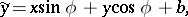which show that the totality of all proper motions on a plane depends on three parameters. The first two parameters characterize the parallel displacement of the plane by the vector, while the parameterdescribes the rotation of the plane around the coordinate origin. A proper motion is the product (composite) of rotation around the coordinate origin through an angleand parallel displacement by a vector. Any proper motion can be represented as a parallel displacement or as a rotation around some point.

Improper motions are expressed by the formulas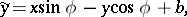which show that an improper motion is the product of a proper motion by a symmetry with respect to some straight line. Any improper motion is the product of a parallel displacement in some direction and a symmetry with respect to a straight line having the same direction.

A proper motion in three-dimensional space is either a rotation around an axis or a parallel displacement, or else can be represented as the product of a rotation around an axis and a parallel displacement in the direction of the axis (helical motion). An improper motion is either a symmetry with respect to a plane or can be represented as the product of a symmetry with respect to a plane and a rotation around an axis normal to that plane, or as the product of a symmetry with respect to a plane by a displacement in the direction of a vector parallel to this plane.

In a rectangular coordinate system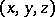in space, the analytic expression of a motion is: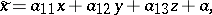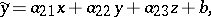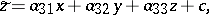where the elements of the matrixsatisfy the following orthogonality conditions: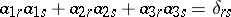(if,if). A motion is proper or improper depending on whether the determinant of this matrix isor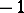. Cf. Orthogonal transformation.

In-dimensional Euclidean spaces a motion is analytically expressed, in a similar manner, in rectangular coordinates, using an orthogonal matrix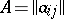, as: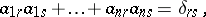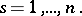A motion of a Riemannian space is a one-to-one continuously-differentiable mapping of class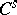,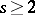, under which the lengths of the respective lines are preserved. The line element of the space,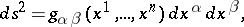where the summation is over the indices, is invariant under a motion. Analytically, a motion is defined by formulas which express the coordinates of a pointafter transformation in terms of the coordinates of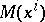, the initial point, using functions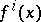of class: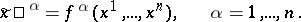(*)

The condition of invariance of the line element means that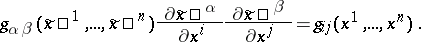The concept of a motion in the Riemannian spaces of the general theory of relativity is of importance: In strong asymmetric gravitational fields solid bodies may become displaced to a very limited extent only; cases in which all motion is impossible may also occur, in which case the metric does not remain invariant under any transformation of space or, in other words, any displacement is accompanied by a deformation of the body.

A motion of a space with an affine connection is a one-to-one continuously-differentiable mapping of class,, under which any field of parallel vectors along any smooth curve becomes a field of parallel vectors of the transformed curve. Under a motion the object of affine connection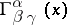(cf. Geometric objects, theory of) is mapped to itself. Conversely, any mapping (*) which maps the affine connection to itself is a motion.

The motions constitute a group of transformations (cf. Group of motions). They are the simplest transformations of a space.

How to Cite This Entry:
Motion. Encyclopedia of Mathematics. URL: http://encyclopediaofmath.org/index.php?title=Motion&oldid=18181
This article was adapted from an original article by I.P. Egorov (originator), which appeared in Encyclopedia of Mathematics - ISBN 1402006098. See original article